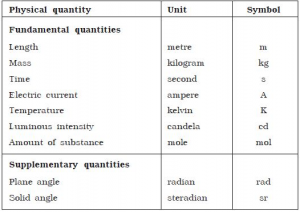# UKPCS Science: Physics- System of Units – Units and Measurements #2

Oracle IAS, the best coaching institute for UPSC/IAS/PCS preparation in Dehradun brings to you UKPCS Science (paper #6).System of Units – Units and Measurements

Introduction

• unit is a particular physical quantity, defined and adopted by convention, with which other particular quantities of the same kind are compared to express their value. A physical quantity is a quantity that can be used in the mathematical equations of science and engineering.
• The value of a physical quantity is the quantitative expression of a particular physical quantity as the product of a number and a unit, the number being its numerical value. Thus, the numerical value of a particular physical quantity depends on the unit in which it is expressed.
• When making measurements, it is customary to record both the quantity (how much) and the unit (of what). Science and technology depend largely on the unit of measurement.
• For example, the value of the height hof building is h = 120 m. Here h is the physical quantity, its value expressed in the unit “meter,” unit symbol m, is 120 m, and its numerical value when expressed in meters is 120.

What are different systems of units?

• A system of units is collection of units in which certain units are chosen as fundamental and all other are derived from them. This system is also called as absolute system of units.
• In most of the system the mass, the length and the time are considered to be fundamental quantities and their units are called as fundamental units.

Following are some system of units which are in common use:
c.g.s. system of units
Unit of length is centimetre (cm). Unit of mass is gram (gm). Unit of time is second (s)
m.k.s. system of units
Unit of length is metre (m). Unit of mass is kilogram (kg). Unit of time is second (s)
f.p.s. system of units
Unit of length is foot (ft). Unit of mass is pound (Lb). Unit of time is second (s)

S.I. system of units:

• In October 1971 a replacement of metric system of units was done with a new system called Systeme Internationale d’Unites.
• This system of units is improvement and extension of the traditional metric system. Now this system has replaced all other systems of units in all branches of science, engineering, industry and technology.

System of Units – Units and MeasurementsCost- Rs.5000/- (including shipping)

(~2500+ pages)

# UKPCS Mains Study Material subject wise

The notes are strictly as per UKPCS syllabus (topic wise):

Individual Polity Cost: Rs. 1500/- (including shipping)

Individual S&T Cost: Rs. 1500/- (including shipping)

Individual Geography Cost: Rs. 1500/- (including shipping)